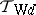# Wijsman convergence

(diff) ← Older revision | Latest revision (diff) | Newer revision → (diff)

R. Wijsman [a4] introduced a convergence for sequences of proper lower semi-continuous convex functions in ${\bf R} ^ { n }$. Workers in topologies on hyperspaces found this convergence and the resulting topology quite useful and subsequently a vast body of literature developed on this topic (see [a1], [a2]).

Suppose $( X , d )$ is a metric space and let $\operatorname{CL} ( X )$ denote the family of all non-empty closed subsets of $X$. For each $x \in X$ and $A \in \operatorname{CL} ( X )$ one sets $d ( x , A ) = \operatorname { inf } \{ d ( x , a ) : a \in A \}$. One says that a net $A _ { \lambda } \in \operatorname{CL} ( X )$ (cf. also Net (of sets in a topological space)) is Wijsman convergent to $A \in \operatorname{CL} ( X )$ if and only if for each $x \in X$, $d ( x , A _ { \lambda } ) \rightarrow d ( x , A )$, i.e. the convergence is pointwise. The resulting topologyon $\operatorname{CL} ( X )$ is called the Wijsman topology induced by the metric $d$. The dependence of the Wijsman topology on the metric $d$ is quite strong in as much as even two different uniformly equivalent metrics may induce different Wijsman topologies. Necessary and sufficient conditions for two metrics to induce the same Wijsman topology have been found by C. Costantini, S. Levi and J. Zieminska, among others. G. Beer showed that if $( X , d )$ is complete and separable (cf. also Complete metric space; Separable space), thenis a Polish space, i.e. it is separable and has a compatible complete metric.

If the pointwise convergence $d ( x , A _ { \lambda } ) \rightarrow d ( x , A )$ is replaced by uniform convergence, then Hausdorff convergence is obtained, which has been known for a long time. The associated Hausdorff topology $\mathcal{T} _ { \text{H}d }$ is derived from the Hausdorff metric $d _ { \text{H} }$ given by $d _ { H } ( A , B ) = \operatorname { sup } \{ | d ( x , A ) - d ( x , B ) | : x \in X \}$). It is known that $T _ { \operatorname{W} d } = T _ { \operatorname{H}d }$ if and only if $( X , d )$ is totally bounded (cf. also Totally-bounded space).

A natural question arises: What is the supremum of the Wijsman topologies induced by the family of all metrics that are topologically (respectively, uniformly) equivalent to $d$. It was shown by Beer, Levi, A. Lechicki, and S. Naimpally that the supremum of topologically (respectively, uniformly) equivalent metrics is the Vietoris topology $T _ { \text{V} }$ (cf. Exponential topology; respectively, the proximal topology $T _ { \delta }$). These are hit-and-miss type topologies; the former has been known for a long time while the latter is a rather recent discovery (1999; cf. also Hit-or-miss topology). It is known that $T _ { W d } = T_{V}$ if and only if $( X , d )$ is compact, while $T _ { \text{W}d } = T _ { \delta }$ is equivalent to $( X , d )$ being totally bounded. G. Di Maio and Naimpally discovered a (hit-and-miss) proximal ball topology $T _ { \text{B} \delta }$ which equals $T_{\text{W}d}$ in almost convex metric spaces (these include normed linear spaces) [a3]. L. Holá and R. Lucchetti have discovered necessary and sufficient conditions for the equality of $T_{\text{W}d}$ and $T _ { \text{B} \delta }$.

The Wijsman topology $T_{\text{W}d}$ is always a Tikhonov topology (cf. also Tikhonov space) and a remarkable theorem of Levi and Lechicki shows that the separability of $X$ is equivalent to $T_{\text{W}d}$ being metrizable or first countable or second countable.

Wijsman's original work has been generalized by U. Mosco, Beer and others. Naimpally, Di Maio and Holá have studied Wijsman convergence in function spaces (see [a2]).

How to Cite This Entry:
Wijsman convergence. Encyclopedia of Mathematics. URL: http://encyclopediaofmath.org/index.php?title=Wijsman_convergence&oldid=51143
This article was adapted from an original article by Som Naimpally (originator), which appeared in Encyclopedia of Mathematics - ISBN 1402006098. See original article